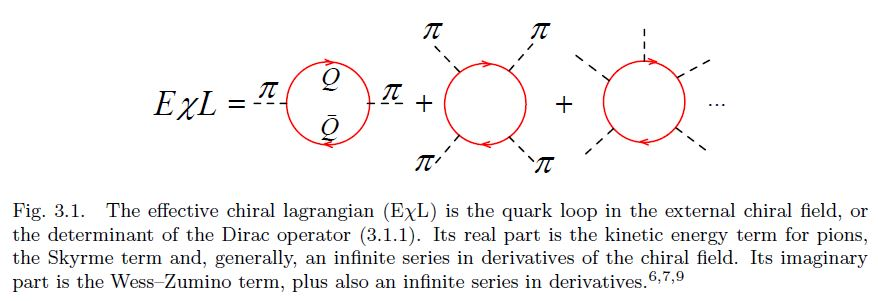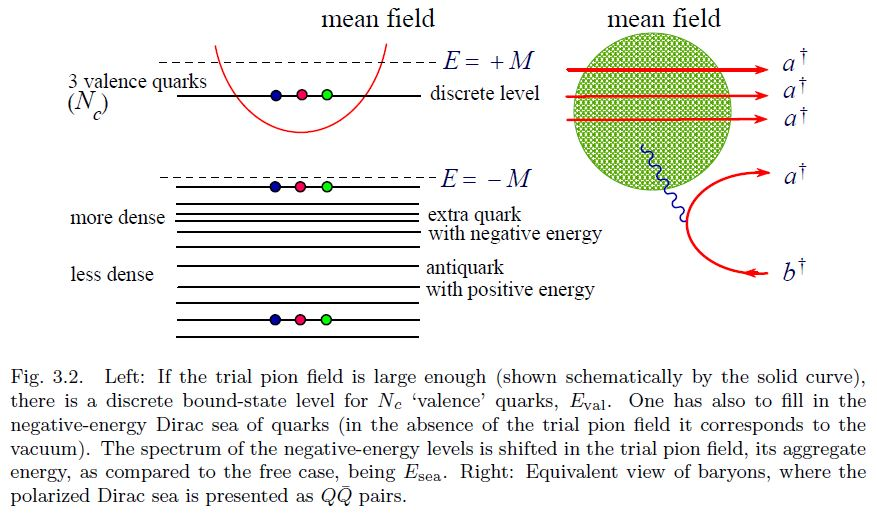# nLab hidden local symmetry

Contents

### Context

#### Fields and quanta

fields and particles in particle physics

and in the standard model of particle physics:

force field gauge bosons

scalar bosons

flavors of fundamental fermions in the
standard model of particle physics:
generation of fermions1st generation2nd generation3d generation
quarks ($q$)
up-typeup quark ($u$)charm quark ($c$)top quark ($t$)
down-typedown quark ($d$)strange quark ($s$)bottom quark ($b$)
leptons
chargedelectronmuontauon
neutralelectron neutrinomuon neutrinotau neutrino
bound states:
mesonslight mesons:
pion ($u d$)
ρ-meson ($u d$)
ω-meson ($u d$)
f1-meson
a1-meson
strange-mesons:
ϕ-meson ($s \bar s$),
kaon, K*-meson ($u s$, $d s$)
eta-meson ($u u + d d + s s$)

charmed heavy mesons:
D-meson ($u c$, $d c$, $s c$)
J/ψ-meson ($c \bar c$)
bottom heavy mesons:
B-meson ($q b$)
ϒ-meson ($b \bar b$)
baryonsnucleons:
proton $(u u d)$
neutron $(u d d)$

(also: antiparticles)

effective particles

hadrons (bound states of the above quarks)

solitons

in grand unified theory

minimally extended supersymmetric standard model

superpartners

bosinos:

dark matter candidates

Exotica

auxiliary fields

# Contents

## Idea

In the effective field theory sigma-models for Goldstone bosons (such as mesons in chiral perturbation theory of QCD) the global symmetry (e.g. chiral symmetry) may typically be enhanced to a “hidden” local gauge symmetry that exhibits the source vector fields (notably: vector mesons) as gauge fields of a (flavour) gauge theory.

In chiral perturbation theory, the gauge bosons of the hidden local chiral symmetry are identified with the vector mesons. The minimal coupling of these two the baryons yields the vector meson dominance-model of quantum hadrodynamics.

In intersecting D-brane models, such as in holographic QCD, this is geometrically brought out by open strings ending on “flavor branes” instead of “color branes”.

## Properties

### Relation to Skyrmions

It is astounding that Skyrme had suggested his model as early as in 1961 before it has been generally accepted that pions are (pseudo) Goldstone bosons associated with the spontaneous breaking of chiral symmetry, and of course long before Quantum Chromodynamics (QCD) has been put forward as the microscopic theory of strong interactions.

The revival of the Skyrme idea in 1983 is due to Witten who explained the raison d’ˆetre of the Skyrme model from the viewpoint of QCD. In the chiral limit when the light quark masses $m_u$, $m_d$, $m_s$ tend to zero, such that the octet of the pseudoscalar mesons π, K , η become nearly massless (pseudo) Goldstone bosons, they are the lightest degrees of freedom of QCD. The effective chiral Lagrangian (EχL) for pseudoscalar mesons, understood as an infinite expansion in the derivatives of the pseudoscalar (or chiral) fields, encodes, in principle, full information about QCD. The famous two-term Skyrme Lagrangian can be understood as a low-energy truncation of this infinite series. Witten has added an important four-derivative Wess–Zumino term to the original Skyrme Lagrangian and pointed out that the overall coefficient in front of the EχL is proportional to the number of quark colours $N_c$.

$[...]$

Soon after Witten’s work it has been realized that it is possible to bring the Skyrme model and the Skyrmion even closer to QCD and to the more customary language of constituent quarks. It has been first noticed $[$6, 7a, 7b, 8$]$ that a simple chiral invariant Lagrangian for massive (constituent) quarks $Q$ interacting with the octet chiral field $\pi_A$ $(A = 1, ..., 8)$,

$\mathcal{L} = \overline{Q} \left( \partial\!\!\!\!/ - M e^{ \tfrac{i \pi^A \lambda^A \gamma_5}{F_\pi} } \right) Q$

induces, via a quark loop in the external pseudoscalar fields (see Fig. 3.1), the EχL whose lowest-derivative terms coincide with the Skyrme Lagrangian, including automatically the Wess–Zumino term, with the correct coefficient!$[...]$

The condition that the winding number of the trial field is unity needs to be imposed to get a deeply bound state, that is to guarantee that the baryon number is unity. $[$9$]$ The Skyrmion is, thus, nothing but the mean chiral field binding quarks in a baryon.effective field theories of nuclear physics, hence for confined-phase quantum chromodynamics:

### General

Origin of effective field theory of mesons in nuclear physics, via hidden local gauge symmetry:

Review:

Further original articles:

Equivalence between hidden local symmetry- and massive Yang-Mills theory-description of Skyrmion quantum hadrodynamics:

• Atsushi Hosaka, H. Toki, W. Weise, Skyrme Solitons With Vector Mesons: Equivalence of the Massive Yang-Mills and Hidden Local Symmetry Scheme, 1988, Z. Phys. A332 (1989) 97-102 (spire:24079)

In view of the chiral anomaly WZW term:

• Michio Hashimoto, Hidden Local Symmetry for Anomalous Processes with Isospin/$SU(3)$ Breaking Effects, Phys. Rev. D54 (1996) 5611-5619 (arXiv:hep-ph/9605422)

With emphasis on Skyrmions involving vector mesons:

• Yong-Liang Ma, Ghil-Seok Yang, Yongseok Oh, Masayasu Harada, Skyrmions with vector mesons in the hidden local symmetry approach, Phys. Rev. D87:034023, 2013 (arXiv:1209.3554)

• Mannque Rho, Skyrmions and Fractional Quantum Hall Droplets Unified by Hidden Symmetries in Dense Matter (arXiv:2109.10059)

### Hadrons as KK-modes of 5d Yang-Mills theory

The suggestion that the tower of observed vector mesons – when regarded as gauge fields of hidden local symmetries of chiral perturbation theory – is reasonably modeled as a Kaluza-Klein tower of D=5 Yang-Mills theory:

That the pure pion-Skyrmion-model of baryons is approximately the KK-reduction of instantons in D=5 Yang-Mills theory is already due to:

with a hyperbolic space-variant in:

The observation that the result of Atiyah-Manton 89 becomes an exact Kaluza-Klein construction of Skyrmions/baryons from D=5 instantons when the full KK-tower of vector mesons as in Son-Stephanov 03 is included into the Skyrmion model (see also there) is due to:

In the Sakai-Sugimoto model of holographic QCD the D=5 Yang-Mills theory of this hadron Kaluza-Klein theory is identified with the worldvolume-theory of D8-flavour branes intersected with D4-branes in an intersecting D-brane model:

Extensive review of this holographic/KK-theoretic-realization of quantum hadrodynamics from D=5 Yang-Mills theory is in:

Via the realization of D4/D8 brane bound states as instantons in the D8-brane worldvolume-theory (see there and there), this relates also to the model of baryons as wrapped D4-branes, originally due to

and further developed in the nuclear matrix model:

In relation to Yang-Mills monopoles:

• Stefano Bolognesi, Solitons, Large $N$ and Holography, 2015 (pdf)

More on baryons in the Sakai-Sugimoto model of holographic QCD:

More on mesons in holographic QCD:

• Daniel Ávila, Leonardo Patiño, Melting holographic mesons by cooling a magnetized quark gluon plasma (arXiv:2002.02470)

• Xuanmin Cao, Hui Liu, Danning Li, Pion quasiparticles and QCD phase transitions at finite temperature and isospin density from holography, Phys. Rev. D 102, 126014 (2020) (arXiv:2009.00289)

• Xuanmin Cao, Songyu Qiu, Hui Liu, Danning Li, Thermal properties of light mesons from holography (arXiv:2102.10946)

• Artur Amorim, Miguel S. Costa, Matti Järvinen, Regge theory in a Holographic dual of QCD in the Veneziano Limit (arXiv:2102.11296)

• R. da Rocha, Information in AdS/QCD: mass spectroscopy of isovector mesons (arXiv:2103.03924)

• Shahin Mamedov, Narmin Nasibova, Temperature dependence of $\rho$ meson-nucleon coupling constant from the soft-wall model (arXiv:2103.10494)

An alternative scenario of derivation of 4d Skyrmions by KK-compactification of D=5 Yang-Mills theory, now on a closed interval, motivated by M5-branes instead of by D4/D8-brane intersections as in the Sakai-Sugimoto model, is discussed in:

following

### In D-brane models

Further interpretation/derivation of hidden local symmetry in holographic QCD: